Mechanics Index

Stress Strain Diagram...

Introduction

The stress-strain diagram is generally accepted as the plotted results of a tensile test completed under carefully controlled conditions on a speciman of a metal.     The stress-strain diagram important for design engineers in that it establishes the physical properties of the material under test including the yield strength, the ultimate strength, the elongation at fracture, the elastic limit etc.    The test is carried out on a bar of uniform cross section, in a testing machine which indicates the tensile load being applied.  The elongation of a calibrated length of the test piece (called the gauge length) is recorded by and extensometer or strain gauge.

The measured load results and the extension results are then converted to stress values (Load/specimen area) and strain values (extension / gauge length ) and the results are plotted on a graph.    The stress levels resulting from this test are nominal or practical engineering values.

The tensile test does not provide any information as to the strength of a material under highly cyclic loading, and does not identify the resistance of the material to shock loading.    Other test procedures are required to test these factors.

Different materials clearly result in different graphs. The notes that follow relate to these different graphs and the important data which is extracted from these graphs.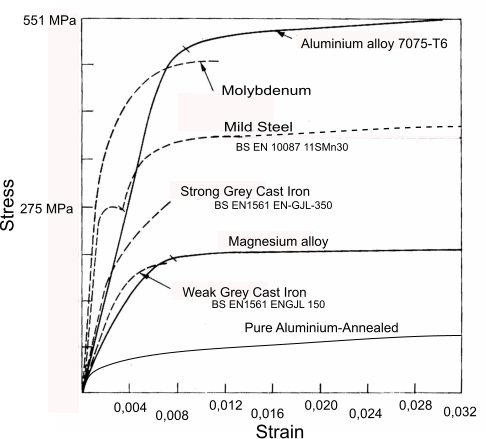Stress Strain Graph showing the curves of some different engineering metals

Notes

This diagram is simply showing some curves for some materials, they are not representative of all the materials of a particular type .  The grey cast irons are shown as representing brittle materials however: ferritic cast irons can have a elongation at failure of 22% (2" gauge length).     The mild steel curve is representative of a metal with a pronounced yield point. There are many alloy steels which are brittle and have tensile strengths far above the range of the graph.

It is also important to identify that the actual physical characteristics of a metal can vary significantly due to its thickness i.e. the proof stress and ultimate stresses quoted in the standards are generally related to the thickness the thicker the section - the lower the average strengths.     The strength /elongation can also be affected by the direction of loading relative to the grain which reflects the direction of rolling/casting.

The stress strain graphs resulting tensile testing are only truly representative of the metal if care is used in the selection of the test specimen and it the evaluation of the curve.

Symbols / Units
 A = % elongation at fracture BS ident. L = length (m) Lo = original gauge length (mm) ) BS ident. Lu = final gauge length (mm) ) BS ident. Lc = length of parallel portion of test piece (mm) ) BS ident. Lt = overall length of test piece (mm) ) BS ident. F = Force (N) σ = Tensile stress (N/m2) E = Young's moudulus of elasticity (MPa) BS ident. ε = strain in direction of stress δ = deflection(m) Fm = maximum force (N) BS ident. mE = Slope of the elastic part of the stress-percentage (MPa) BS ident ReH = upper yield strength (MPa) BS ident. ReL = lower yield strength (MPa) BS ident. Rm = tensile strength (MPa) BS ident. Rp = proof strength, plastic extension (MPa) BS ident. Rr = Specified permanent set strength (MPa) BS ident. Rt = MPa proof strength,total extension (MPa) BS ident. So = original cross section area of test piece (mm2) BS ident. Su = minimum cross section area after fracture (mm2) BS ident. S = Current minimumum cross section area of test piece (mm2) BS ident.

Reference Standard

BS EN ISO 6892 - 1:2009 Metallic Materials Tensile Testing. Part 1 Method of Test at Room Temperatures

Engineering Stress Strain Diagram for Mild Steel

The diagram below shows the stress strain diagram for a typical ductile steel.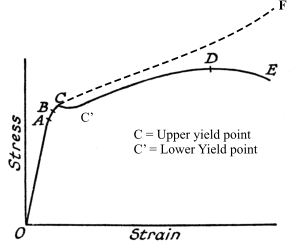The important points on this graph are identified as follows.

The stress strain relationship is a straight line between 0 and A . This region is called the Elastic region. Within this region if the load is removed the specimen would return to its original length.    The slope of the curve in this region (stress/strain) is identified as Youngs Modulus (E).    The design of machines is based on component materials operating within this region.     The point A is called the Elastic/Proportional limit.

The region from A to the breaking point E /F the region is called the plastic region.

Between A and B some plastic yielding takes place . Removal of the stress results in significant recovery of the initial length.

At point C there is a definite extension with no increase in load.    This is called the yield point and is primarily exhibited in ductile materials such as mild steel.  The stress reduces at C and there are two yield stress values used to identify the yield value i.e. the upper yield point and the lower yield point.    For materials exhibiting no clear yield point an value proof stress is identified as the point of onset of yielding e.g. a 0,2% proof stres is the point at with the material has yielded (non-recoverable extension) by 0,2%.

Between C and D the specimen continues to extend as the load increases the extension being primarily not recoverable the material is deforming plastically.    Removing the load will result in recovery of only the strain between 0 and A.    The material is actually becoming a little stronger in this region as a result of cold working

At point D the specimen will exhibit a significant reduction is section diameter within the calibration length.  The specimen will start to neck.  The reference ultimate /maximum tensile stress of a material is calculated by dividing the load by the original section area.

In the region from C to D the diameter of the specimen is reducing as the linear strain increases. this is a result of the Poissen effect.    From D to E the cross section will reduce significantly as the specimen approaches failure.    The true stress in the region from C to Fracture is shown as the dotted line and, is different to that recorded on the stress-strain curve. The true stress is shown on the diagram as the curve from C to F.

From D to E the specimen will start to lengthen with no increase in load and the overall strain at point E is important as it identifies the ductility of the material and is listed in the material standards as the % elongation at rupture.

Note:
It is important to note that the % elongation at failure is not a real value. The result from the test measures the elongation over "The Gauge Length".  In fact most of the real elongation only occurs over a short length due to localised necking.    Thus the elongation effect over a 50mm gauge length may be 20% , Over a 100mm gauge length the elongation would probable be measured at near 10%"

Stress Strain Diagram brittle Material

The diagram below shows Stress strain graph for a brittle and a ductile material.    A typical brittle material is Cast Iron.    Steels become increasingly brittle as the carbon content increases.

Brittle fractures are generally clean and the ends of the test piece are sqaure and mate together.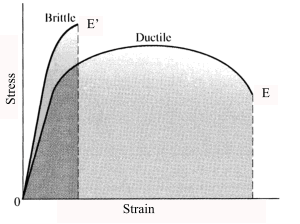True Stress and Strain

It is clear that the tensile testing procedure yields important values for specifying engineering materials.    It is however important to note that the stress and strain values identified in the test are not true stress and strain values.    Thery are known as Engineeering stress and engineering strain.

Engineering stress = Force / Original Specimen Area = F / So
Engineering strain = extension/ Original Specimen Length = ( Lu - Lo ) / Lo

The true stress and the true strain differs from the real value continuously as the strain increases.

The true stress = Force / Actual Specimen minimum area Area = F / S
True strain = logarithmic strain = Sum of incremental elongations /current length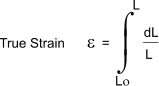Tensile Test Specimen

The diagram below shows test specimen geometry as illustrated in the above identified standard. The test specimen can be flat, round, rectangular, hexagonal,or tubular. The geometry of the test specimen is selected based on the material being tested i.e a round bar would require a round test piece, a sheet would require a flat test piece.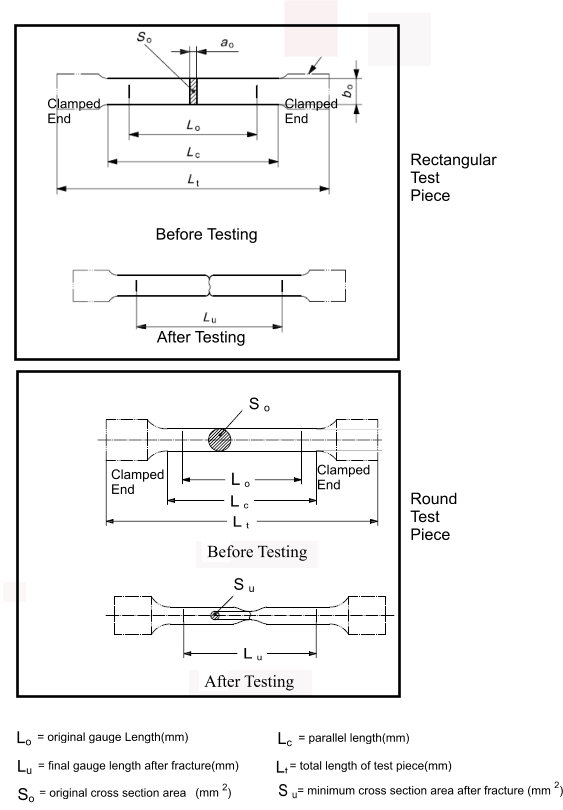Typical proportions for a round test piece are

d = 5mm, Lo = 25mm, Lc = 28mm
d = 10mm, Lo = 50mm, Lc = 55mm
d = 14mm, Lo = 70mm, Lc = 77mm
d = 20mm, Lo = 100mm, Lc = 10mm

 Links to Dynamics /Kinetics Stress Strain Curve Wikipedea;... An excellent treatment of the topic..

Mechanics Index